# Show all steps 1. (10 points) Find the Laplace transform of the following functions a. f(t)...

Show all steps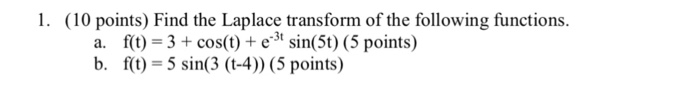1. (10 points) Find the Laplace transform of the following functions a. f(t) 3+cos(t) e3t sin(5t) (5 points) b. f() 5sin(3 (t-4) (5 points)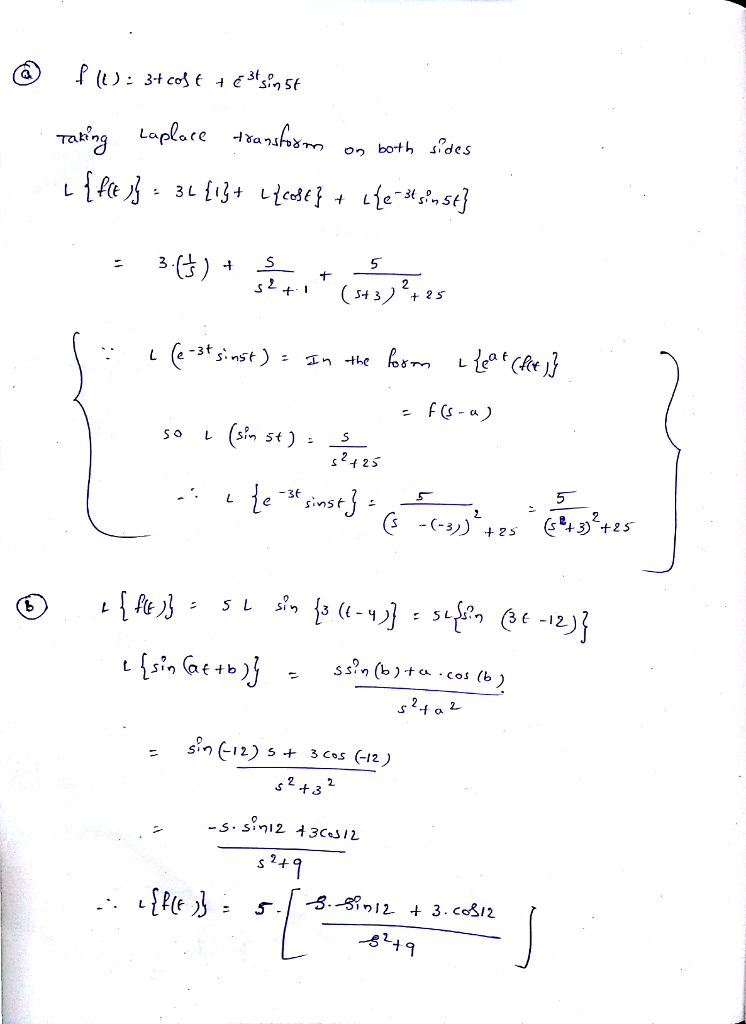##### Add Answer to: Show all steps 1. (10 points) Find the Laplace transform of the following functions a. f(t)...
Similar Homework Help Questions
• ### Calculate the Laplace transform of the following time functions by applying the Laplace transform properties: f)...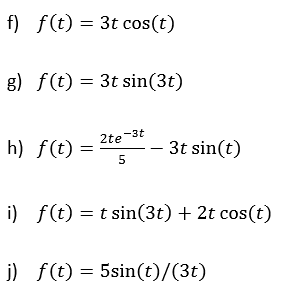Calculate the Laplace transform of the following time functions by applying the Laplace transform properties: f) f(t) = 3t cos(t) g) f(t) = 3t sin(3t) h) f(t) = 2te*** – 3t sin(t) i) f(t) = t sin(3t) + 2t cos(t) j) f(t) = 5sin(t)/(3t)

• ### Find the Laplace transform of each of the following functions. 1. \$(t) = f*(4(t – 1)*...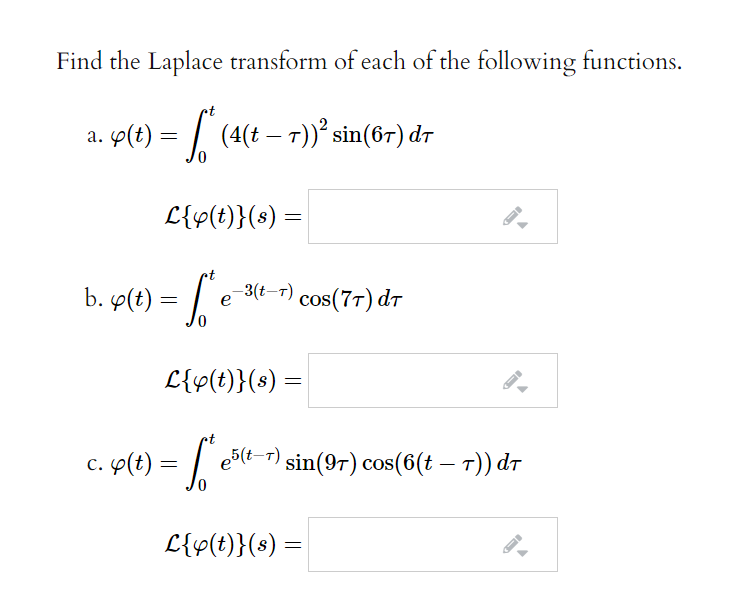Find the Laplace transform of each of the following functions. 1. \$(t) = f*(4(t – 1)* sin(67) dt L{v(t)}(s) = b. g(t) = [ e 2-3(t-1) cos(71) dT L{\$(t)}(s) = c. y(t) = e5(t-1) sin(97) cos(6(t – T)) dt L{s(t)}(s) =

• ### 2. (8 points) Find the Laplace transform of each of the following functions. 1. 2 f(t)...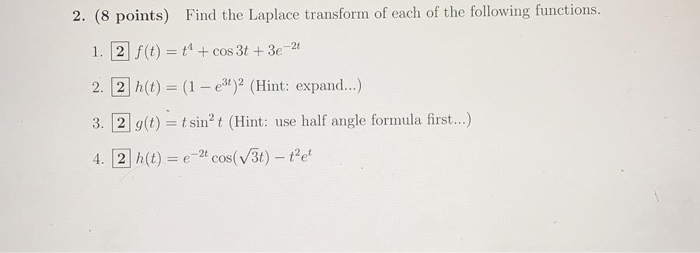2. (8 points) Find the Laplace transform of each of the following functions. 1. 2 f(t) = +" + cos 3t + 3e-21 2. 2h(t) = (1 - 236)(Hint: expand...) 3. 2 g(t) = t sin’t (Hint: use half angle formula first...) 4. 2 h(t) = e-2 cos(/3t) – tet

• ### 2. (8 points) Find the Laplace transform of each of the following functions. 1. 2 f(t)...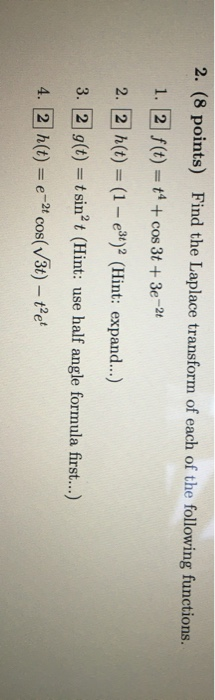2. (8 points) Find the Laplace transform of each of the following functions. 1. 2 f(t) = 14 + cos 3t + 3e-2t 2. 2 h(t) = (1 - 3t)? (Hint: expand...) 3. 2 g(t) = t sin’t (Hint: use half angle formula first...) 4. 2 h(t) = e-2 cos(v3t) - tet

• ### SHOW ALL YOUR WORK TO RECEIVE FULL CREDIT. 1. (2 points) Using the definition, find the...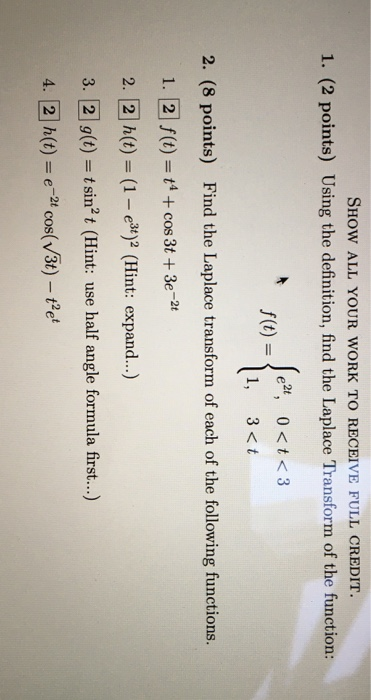SHOW ALL YOUR WORK TO RECEIVE FULL CREDIT. 1. (2 points) Using the definition, find the Laplace Transform of the function: e2t, 0 <t <3 f(t) = { 1, 3<t 2. (8 points) Find the Laplace transform of each of the following functions. 1. 2 f(t) = 14 + cos 3t + 3e-2 2. 2 h(t) = (1 - e3t)2 (Hint: expand...) 3. 2 g(t) = t sin’t (Hint: use half angle formula first...) 4. 2 h(t) = e-2 cos(v3t)...

• ### is 45-5 The inverse Laplace transform of F(s) = \$?+9 Select the correct answer a. 4cos3t...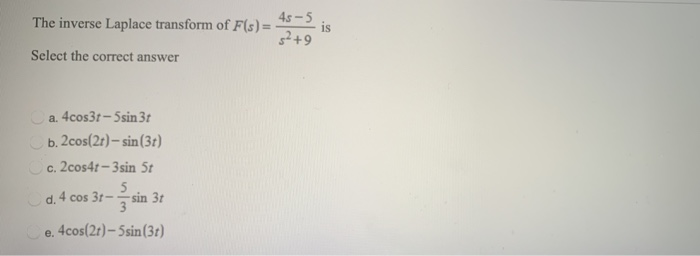is 45-5 The inverse Laplace transform of F(s) = \$?+9 Select the correct answer a. 4cos3t - 5sin 31 b. 2cos(2t) - sin (36) c. 2cos4 - 3sin 5t 5 d. 4 cos 3-sin 31 e. 4cos(26) - 5sin (31)

• ### Q1) Find the Laplace Transform of the following functions: 1. e +5 2. cos(2t)+7sin(2) 3t)+sin(3) 4....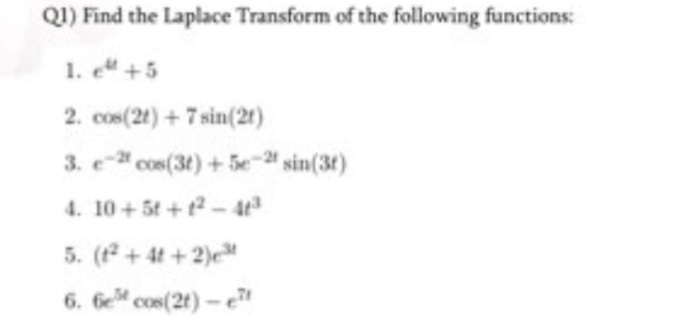Q1) Find the Laplace Transform of the following functions: 1. e +5 2. cos(2t)+7sin(2) 3t)+sin(3) 4. 10+ 5t +12-4 5. (+2)e 6. Gcos(21)-

• ### Q2.(10 ts) Find the Laplace transform of the following. Show all work 1.f(t) = t? cost...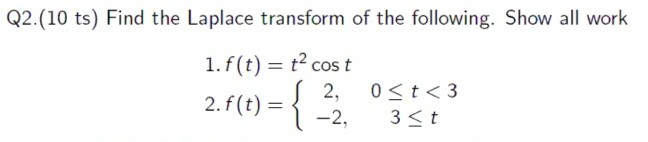Q2.(10 ts) Find the Laplace transform of the following. Show all work 1.f(t) = t? cost 2.f(t) = { 2, Ost<3 3<t

• ### B. Find the Laplace transform of the following functions. 1. f(t) = {" where n is...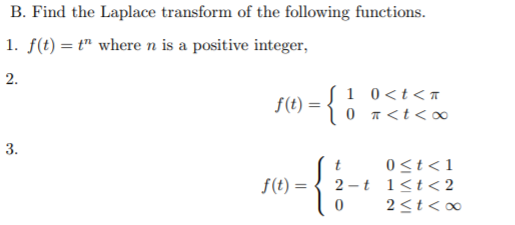B. Find the Laplace transform of the following functions. 1. f(t) = {" where n is a positive integer, 2. f(t) = { • 10<t< 0 <t< 3. f(t) = { t 0<t<1 2-t i<t<2 0 2<t<

• ### Find the Laplace transforms of the following functions: a) f(t) = sin(at + b)   Using the...Find the Laplace transforms of the following functions: a) f(t) = sin(at + b)   Using the integral of the Laplace transform b) f(t) = cos(t) + sin(t/2)   You can directly use table 5.1 Tableau 5.1 Transformées de Laplace les plus couramment utilisées f(t)= £. {F()} F(s)= £{f(t)} f(t)=1 F(s) = 2 f(t)=1 F(s) == 2 3 Sl)=12 F(s) n! 4 St=1" F(s)=- 5 () at F(s)- S-a n! 6 S()=1"ar F(\$)= (s-a)"+1 a 7 s(t)= sin(at) F(s) s? +a? S...

Free Homework App